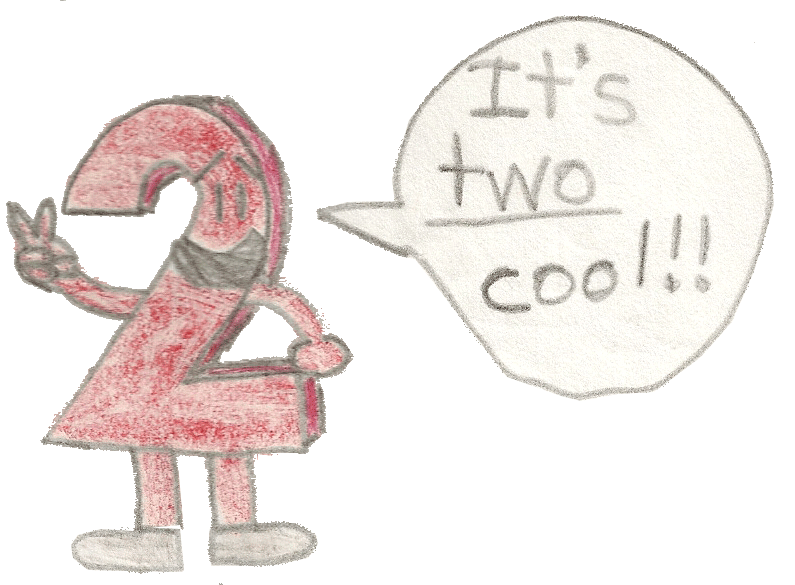# The Halves of Odd Numbers: A Technique of Simplification

## Examples:

59/2 = (59 - 1)/2 + ½ = 58/2 + ½ = 29½

13/2 = (13 - 1)/2 + ½ = 12/2 + ½ = 6½

27/2 = (27 - 1)/2 + ½ = 26/2 + ½ = 13½

121/2 = (121 - 1)/2 + ½ = 120/2 + ½ = 60½

4,321/2 = (4,321 - 1)/2 + ½ = 4,320/2 + ½ = 2,160½

-35/2 = -(35 - 1)/2 - ½ = -34/2 - ½ = -17½

If the number you pick is negative, put that minus sign outside of the parentheses to the left & subtract ½ instead of adding, because negative numbers work backwards in addition!

Better yet, you can simply pick the additive inverse of a negative number & as the final step, multiply by -1.

-(35/2) = -((35 - 1)/2 + ½) = -(34/2 + ½) = -(17½) = -17½

Actually, you can still get the correct answer if you simply plug it into x:

(-35)/2 = (-35 - 1)/2 + ½) = -36/2 + ½) = -18 + ½ = -17½

## And as you know, dividing a number by 2 gives you the number's half!